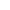# Find the exponential function f(x)=axBest Answer pan.gejt , 23 February 2020 - 07:45 PM

Solutions of these problems are similar to your previous question

https://community.ca...orm/#entry63081

I see 3 solutions. Let's suppose the exp function in following form: y=a×bx

The points are

a. [3,64] and [0,1]
b. [3,343] and [0,1]

1. using direct computing,
so
1=a×b0
64=a×b3

similarly
1=a×b0
343=a×b3

1=a×b=>a=1
64=1×b=>b=4 (by using the 3rd root)
so the equation is
y=4

343=1×b=>b=7 (by using the 3rd root)
so the equation is
y=7
--------------------------------------------------------------------

2. Using exp regression

Switch your calc to Statistics menu (menu - statistics) and input x-values to List 1 and y-values to List 2 (If you have in List 1 and List 2 any data, use another number of the List - e.g. List 3 and List 4, but donť mix x-values with y-values)

Press F6 (command SET) and assign the lists, where you stored the data, to 2Var XList and 2Var YList.
If you don't see the set command, you are probably on the main screen (graph, calc, test, intr, dist) - in such case press F2 (calc) and continue with the steps above.

Set 2Var Freq as 1.

Press exit, press F3 (command REG), F6 (arrow), F2 (command exp) and F2 (ab) and you will see the results:

a = 1
b = 4
resp.
a = 1
b = 7

the equation is: y = 4x  , resp. y=7
--------------------------------------------------------------------

3. Write a small program

"X="?->A:"Y="?->B:Locate 1,1,"B=":Locate 3,1,A(n-th root)B

to get the n-th root press shift + n-th power (between xand exit)

Go to the full post### #1procode200

procode200

Newbie

•• Validating
•• 13 posts
• Gender:Not Telling

Posted 22 February 2020 - 06:45 PM

Does anyone know how to do problems like this (using one point or two points) on the fx-cg50?

Find the exponential function f (x) = ax that passes through the point (3,64) and has a y-intercept of 1

Find the exponential function f (x) = ax that passes through the point (3,343) and has a y-intercept of 1

Edited by procode200, 22 February 2020 - 06:51 PM.

### #2pan.gejt

pan.gejt

Casio Freak

•• Members
•• 253 posts
• Gender:Male
• Location:CZ

• Calculators:
.

Posted 23 February 2020 - 07:45 PM   Best Answer

Solutions of these problems are similar to your previous question

https://community.ca...orm/#entry63081

I see 3 solutions. Let's suppose the exp function in following form: y=a×bx

The points are

a. [3,64] and [0,1]
b. [3,343] and [0,1]

1. using direct computing,
so
1=a×b0
64=a×b3

similarly
1=a×b0
343=a×b3

1=a×b=>a=1
64=1×b=>b=4 (by using the 3rd root)
so the equation is
y=4

343=1×b=>b=7 (by using the 3rd root)
so the equation is
y=7
--------------------------------------------------------------------

2. Using exp regression

Switch your calc to Statistics menu (menu - statistics) and input x-values to List 1 and y-values to List 2 (If you have in List 1 and List 2 any data, use another number of the List - e.g. List 3 and List 4, but donť mix x-values with y-values)

Press F6 (command SET) and assign the lists, where you stored the data, to 2Var XList and 2Var YList.
If you don't see the set command, you are probably on the main screen (graph, calc, test, intr, dist) - in such case press F2 (calc) and continue with the steps above.

Set 2Var Freq as 1.

Press exit, press F3 (command REG), F6 (arrow), F2 (command exp) and F2 (ab) and you will see the results:

a = 1
b = 4
resp.
a = 1
b = 7

the equation is: y = 4x  , resp. y=7
--------------------------------------------------------------------

3. Write a small program

"X="?->A:"Y="?->B:Locate 1,1,"B=":Locate 3,1,A(n-th root)B

to get the n-th root press shift + n-th power (between xand exit)

Edited by pan.gejt, 23 February 2020 - 07:46 PM.

#### 0 user(s) are reading this topic

0 members, 0 guests, 0 anonymous users